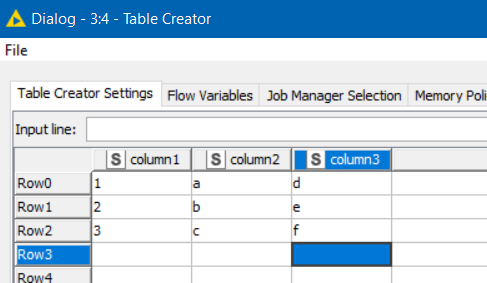# Write to Table Creator Option

It would be great if we could populate the Table Creator node with an optional input. It would allow us to quickly populate table creator nodes for easy portability in KNIME Forum Solutions.

It would also allow us built in read / write file locations within component folders that we could use as log files.

Yes I confess that the Table Creator is probably my least favourite node from the usability side. As an aside… pasting in data is mostly ok, but an option to paste in the Column Names, and a less clunky way to set the data types would be great. That was the driver for me writing the Extract Data Table from Delimited Text component, but the downside of adding a component in answer to a forum question is it can add confusion especially to a newer user.

Re your idea… If the Table Creator had an optional input, I guess the challenge would be how manual entry would affect the data that had been read in. Would it remember the edits you’d made, and what does it do with any manual entry if the input file data changes?

In a related thought, I’d like there to be a “Table Creator Settings Writer” node that allows us to send any table output to a file (just like we do for Excel, CSV etc) except that this file could then be used with the File-Load Settings menu on a Table Creator.

An alternative would be that the “Save Settings” menu option on the Table Creator could be added as a menu option on the View Port dialog, so that on inspecting any data output it would be possible to save that data table as a Table Creator settings file… It may sound a little mad (in a “why would you want to do that?” way), but I actually think there are times when that would prove useful.

Maybe one day I’ll spend time hacking together a component build a settings file, but the xml is not nice even for a simple table like this:.

``````<?xml version="1.0" encoding="UTF-8"?>
<config xmlns="http://www.knime.org/2008/09/XMLConfig" xmlns:xsi="http://www.w3.org/2001/XMLSchema-instance" xsi:schemaLocation="http://www.knime.org/2008/09/XMLConfig http://www.knime.org/XMLConfig_2008_09.xsd" key="dialog">
<config key="internal_node_subsettings">
<entry key="memory_policy" type="xstring" value="CacheSmallInMemory"/>
</config>
<config key="model">
<config key="rowIndices">
<entry key="array-size" type="xint" value="9"/>
<entry key="0" type="xint" value="0"/>
<entry key="1" type="xint" value="0"/>
<entry key="2" type="xint" value="0"/>
<entry key="3" type="xint" value="1"/>
<entry key="4" type="xint" value="1"/>
<entry key="5" type="xint" value="1"/>
<entry key="6" type="xint" value="2"/>
<entry key="7" type="xint" value="2"/>
<entry key="8" type="xint" value="2"/>
</config>
<config key="columnIndices">
<entry key="array-size" type="xint" value="9"/>
<entry key="0" type="xint" value="0"/>
<entry key="1" type="xint" value="1"/>
<entry key="2" type="xint" value="2"/>
<entry key="3" type="xint" value="0"/>
<entry key="4" type="xint" value="1"/>
<entry key="5" type="xint" value="2"/>
<entry key="6" type="xint" value="0"/>
<entry key="7" type="xint" value="1"/>
<entry key="8" type="xint" value="2"/>
</config>
<config key="values">
<entry key="array-size" type="xint" value="9"/>
<entry key="0" type="xstring" value="1"/>
<entry key="1" type="xstring" value="a"/>
<entry key="2" type="xstring" value="d"/>
<entry key="3" type="xstring" value="2"/>
<entry key="4" type="xstring" value="b"/>
<entry key="5" type="xstring" value="e"/>
<entry key="6" type="xstring" value="3"/>
<entry key="7" type="xstring" value="c"/>
<entry key="8" type="xstring" value="f"/>
</config>
<entry key="rowIdPrefix" type="xstring" value="Row"/>
<entry key="rowIdSuffix" type="xstring" value=""/>
<entry key="rowIdStartValue" type="xint" value="0"/>
<entry key="highlightOutputTable" type="xboolean" value="false"/>
<config key="columnProperties">
<config key="0">
<entry key="UserSetValues" type="xboolean" value="false"/>
<entry key="MissValuePattern" type="xstring" value=""/>
<entry key="FormatParameter" type="xstring" isnull="true" value=""/>
<entry key="SkipThisColumn" type="xboolean" value="false"/>
<entry key="ColumnName" type="xstring" value="column1"/>
<config key="ColumnClass">
<entry key="cell_class" type="xstring" value="org.knime.core.data.def.StringCell"/>
<entry key="is_null" type="xboolean" value="false"/>
</config>
</config>
<config key="1">
<entry key="UserSetValues" type="xboolean" value="false"/>
<entry key="MissValuePattern" type="xstring" value=""/>
<entry key="FormatParameter" type="xstring" isnull="true" value=""/>
<entry key="SkipThisColumn" type="xboolean" value="false"/>
<entry key="ColumnName" type="xstring" value="column2"/>
<config key="ColumnClass">
<entry key="cell_class" type="xstring" value="org.knime.core.data.def.StringCell"/>
<entry key="is_null" type="xboolean" value="false"/>
</config>
</config>
<config key="2">
<entry key="UserSetValues" type="xboolean" value="false"/>
<entry key="MissValuePattern" type="xstring" value=""/>
<entry key="FormatParameter" type="xstring" isnull="true" value=""/>
<entry key="SkipThisColumn" type="xboolean" value="false"/>
<entry key="ColumnName" type="xstring" value="column3"/>
<config key="ColumnClass">
<entry key="cell_class" type="xstring" value="org.knime.core.data.def.StringCell"/>
<entry key="is_null" type="xboolean" value="false"/>
</config>
</config>
</config>
</config>
</config>
``````

so I think I’m going to have to be very bored before embarking on it2 Likes

I guess that I was kind of picturing it with an “optional input”. If it is connected, then the incoming data would overwrite the Table Creator on every execution. If it is disconnected, then the Table Creator node would operate as normal sot that manual edits could be made and stored.

That way we effectively can load it with Column Names / Datatypes / Data by just connecting it to any node’s outputs. Then disconnect it and have a portable / manually editable file.

1 Like